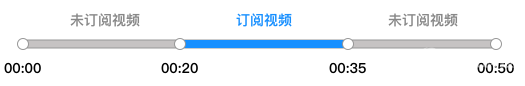计费类型 用户 订阅 视频实际接收分辨率 单价（元/千分钟） 音频 - 10.00 视频 标清（SD） 不高于640 × 480（含） 20.00

TRTC 会根据用户实际接收到的视频分辨率划分视频档位，然后分别对不同档位的视频时长进行计费。A 产生的用量及费用：
A 产生的费用 = A 接收 B 的费用 = 标清视频时长单价 × 标清时长 =20/千分钟 × (30分钟 / 1000) = 0.6

B 产生的用量及费用：
B 产生的费用 = B 接收 A 的费用 = 标清视频时长单价 × 标清时长 = 20/千分钟 × (30分钟 / 1000) = 0.6

 发送方接收方 A640 × 360（标清） B纯音频 C640 × 360（标清） 备注 A - ✓ ✓ 发送视频，订阅 B 的音频，订阅 C 的视频 B ✓ - ✓ 发送音频，订阅 A 的视频，订阅 C 的视频 C ✓ ✓ - 发送视频，订阅 A 的视频，订阅 B 的音频

A 产生的用量及费用：A 产生的费用 = A 接收 B 的费用 + A 接收 C 的费用

A 接收 B 的费用 = 语音时长单价 × 语音时长 = 10/千分钟 × (30分钟 / 1000= 0.3

A 接收 C 的费用 = 标清视频时长单价 × 标清时长 = 20/千分钟 × (30分钟 / 1000) = 0.6

A 产生的费用为 A 接收 B 的费用 + A 接收 C 的费用 = 0.3+ 0.6 = 0.9

B 产生的用量及费用：B 产生的费用 = B 接收 A 的费用 + B 接收 C 的费用

B 接收 A 的费用 = 标清视频时长单价 × 标清时长 = 20/千分钟 × (30分钟 / 1000) = 0.6

B 接收 C 的费用 = 标清视频时长单价 × 标清时长 = 20/千分钟 × (30分钟 / 1000) = 0.6

B 产生的费用为 = B 接收 A 的费用 + B 接收 C 的费用 = 0.6+ 0.6 = 1.2

C 产生的用量及费用：C 产生的费用 = C 接收 A 的费用 + C 接收 B 的费用

C 接收 A 的费用 = 标清视频时长单价 × 标清时长 = 20/千分钟 × (30分钟 / 1000) =0.6

C 接收 B 的费用 = 语音时长单价 × 语音时长 = 10/千分钟 × (30分钟 / 1000= 0.3

C 产生的费用为 = C 接收 A 的费用 + C 接收 B 的费用 = 0.6 + 0.3 =0.9